Function Repository Resource:

# BialternateProduct

Calculate the bialternate product of two square matrices

Contributed by: Jan Mangaldan
 ResourceFunction["BialternateProduct"][m1,m2] constructs the bialternate product of the square matrices m1 and m2.

## Details

The bialternate product of matrices m1 and m2 is a matrix whose entries are half the sums of determinants of 2×2 matrices formed from taking rows and columns of the two matrices in pairs.
The square matrices m1 and m2 must have the same dimensions.
If m1 and m2 are both of length n, then the bialternate product is a square matrix of length n(n-1)/2.

## Examples

### Basic Examples (1)

Compute the bialternate product of two symbolic 3×3 matrices:

 In:=Out=### Scope (2)

Compute the bialternate product of two exact matrices:

 In:=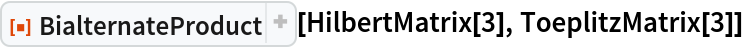Out=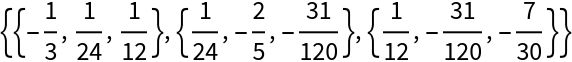Compute the bialternate product of two numerical matrices:

 In:=Out=### Properties and Relations (4)

The bialternate product is multilinear (linear in each argument):

 In:=In:=Out=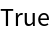In:=Out=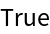The bialternate product is commutative:

 In:=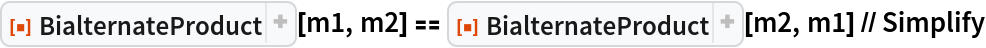Out=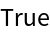Transposition distributes over the bialternate product:

 In:=Out=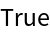The eigenvalues of the bialternate product of a matrix with itself are the products of the eigenvalues of the original matrix, taken in pairs:

 In:=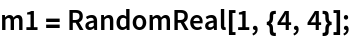In:=In:=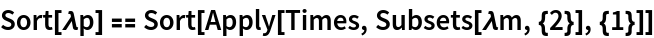Out=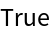The bialternate product of a matrix with twice the identity matrix of the same dimension is the bialternate sum of a matrix:

 In:=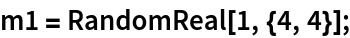In:=Out=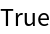The eigenvalues of the bialternate sum are the sums of the eigenvalues of the original matrix, taken in pairs:

 In:=In:=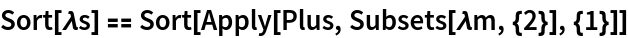Out=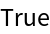## Version History

• 1.0.0 – 11 April 2022